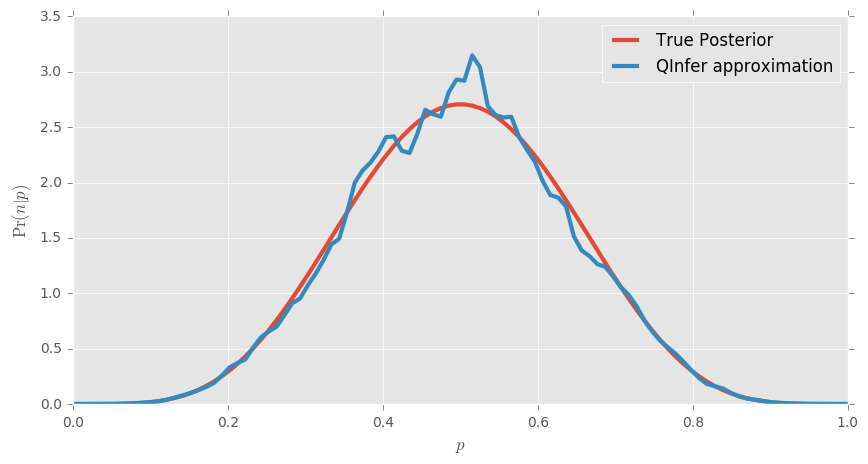# Lecture 5: Data Analysis with QInfer (1.5 hours)¶

### ABSTRACT¶

We will now introduce Bayesian parameter estimation and the sequential Monte Carlo algorithm as implemented by QInfer. The intent is not to have a deep understanding of the general problem of parameter estimation at the end of this, but to get a flavor of how to use what you have learned in the previous lectures to utilize a mature, but specialized Python package. Part of the goal will be for you to see analogies to these examples in the statistical problems you might be facing in your own research, and to have a new Pythonic solution. (Also, at the end you should be excited and eager to use Bayesian inference for all your statistical needs!)

In this Lecture you will learn how to:

• estimate the bias of a classical coin,
• use QInfer to perform:
• quantum state tomography,
• phase estimation,
• randomized benchmarking,
• specify custom models to use with QInfer.

### Preamble¶

Here we import all the necessary modules as well as make the code compatible with Python 2.7:

In :
from __future__ import division, print_function

import qinfer as qi
import numpy as np
import scipy as sp
import matplotlib.pyplot as plt

%matplotlib inline
# Use nice plotting defaults if available.
try: plt.style.use('ggplot')
except: pass


## Starting simple: estimating the bias of a coin¶

Suppose we have a coin that we do not know the bias $p$ of. We will guess what $p$ is by flipping the coin many times.

If we knew the bias, we could determine the exact distribution of the number of "Heads" we would see. If we label the number of flips $N$ and the number of heads $n$, then the distribution of $n$ is a Binomial distribution: $$\operatorname{Pr}(n|p) = \binom{N}{n} p^n (1-p)^{N-n}.$$

Now suppose we have flipped the coin and $n$ heads did actually occur in $N$ flips. What is the distribution of $p|n$? The answer is given by Bayes' rule: $$\operatorname{Pr}(n|p) = \frac{\operatorname{Pr}(n|p)\operatorname{Pr}(p)}{\operatorname{Pr}(n)},$$ where $\operatorname{Pr}(n|p)$ is called the likelihood function, $\operatorname{Pr}(p)$ the prior, $\operatorname{Pr}(p|n)$ the posterior and $\operatorname{Pr}(n)$ is called the evidence. The likelihood function we have determined above. The prior is the input to the problem and the evidence can be determined by normalization of probability.

The input, the prior, can be anything. A typical choice for generic problems is the uniform prior $\operatorname{Pr}(p) = 1/V$, where $V$ is the volume of the parameter space, in this case $V = 1$ since the bias can be between 0 and 1. For the uniform prior, the posterior is $$\operatorname{Pr}(p|n) = \frac{p^n (1-p)^{N-n}}{B(n+1,N-n+1)},$$ where $B$ is the Beta function. (See a derivation of a slightly more general form here).

### Flipping coins¶

Suppose we flip our coin 10 times. What does $\operatorname{Pr}(n|p)$ look like?

In :
# Create a variable for the number of flips (go ahead and change this if you want).
N = 10
# This creates an array containing [0, 1, ..., N].
n = np.arange(0, N + 1)

# This sets up the figure as an matrix of subfigures with 1 row and 3 columns.
fig, axarr = plt.subplots(1, 3, figsize=(15, 5))
# This is the probability mass function (pmf) of the binomial distribution for p = 0.
dist = sp.stats.binom.pmf(n, N, 0)
# On the first (0 indexed!) subplot, make a bar plot with x-axis given by the array n and y-axis given by the pmf.
axarr.bar(n, dist)

# The rest is just annotation and repeating for different biases.
axarr.set_xlabel("$n$")
axarr.set_ylabel("$\operatorname{Pr}(n|p)$")
axarr.set_title("$p=0$")

dist = sp.stats.binom.pmf(n, N, 0.1)
axarr.bar(n, dist)
axarr.set_xlabel("$n$")
axarr.set_title("$p = 0.1$")

dist = sp.stats.binom.pmf(n, N, 0.5)
axarr.bar(n, dist)
axarr.set_xlabel("$n$")
axarr.set_title("$p = 0.5$")

Out:
<matplotlib.text.Text at 0xdf0d160>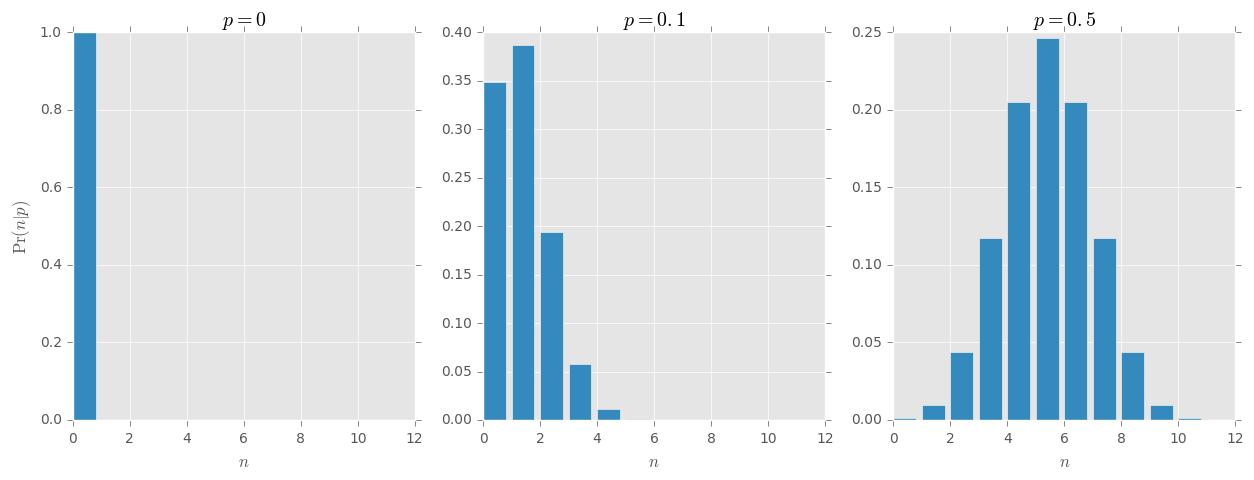In reality, we often have data, in this case $n$, so we are interested not in $\operatorname{Pr}(n|p)$ but in $\operatorname{Pr}(p|n)$. We still use $\operatorname{Pr}(n|p)$ in Bayes' rule but think of it as a function of $p$ for fixed data $n$. Below we plot what that looks like.

In :
 # This creates an array with 100 values between 0 and 1.
p = np.linspace(0, 1, 100)

fig, axarr = plt.subplots(1, 3, figsize=(15, 5))
likelihood = sp.stats.binom.pmf(0, N, p)
axarr.plot(p, likelihood, linewidth=3)
axarr.set_xlabel("$p$")
axarr.set_ylabel("$\operatorname{Pr}(n|p)$")
axarr.set_title("$n = 0$")

likelihood = sp.stats.binom.pmf(1, N, p)
axarr.plot(p, likelihood, linewidth=3)
axarr.set_xlabel("$p$")
axarr.set_title("$n = 1$")

likelihood = sp.stats.binom.pmf(5, N, p)
axarr.plot(p, likelihood, linewidth=3)
axarr.set_xlabel("$p$")
axarr.set_title("$n = 5$")

Out:
<matplotlib.text.Text at 0x10625470>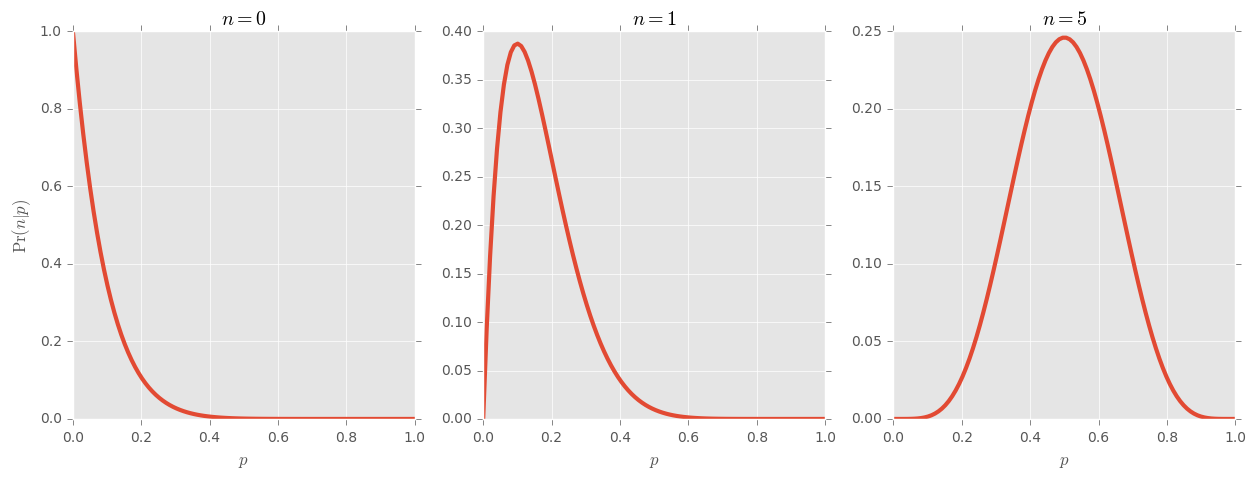Bayes' rule states: $$\operatorname{Pr}(p|n) = \frac{\operatorname{Pr}(n|p)\operatorname{Pr}(p)}{\operatorname{Pr}(n)} = \frac{p^n (1-p)^{N-n}}{B(n+1,N-n+1)},$$ For the uniform prior $\operatorname{Pr}(p) = 1$. Since this is just proportional to $\operatorname{Pr}(p|n)$ we should expect the posterior to look the same.

(Note that $\operatorname{Pr}(p|n)$ is not strictly a probability, but a density, or measure, and can be greater than 1 for any particular value of $p$ since only questions about the probability over intervals return non-zero answers.)

In :
# Rather than write it out many times, we define a function to compute the posterior which compresses further code.
def posterior(p, n, N):
return p ** n * (1 - p) ** (N - n) / sp.special.beta(n + 1, N - n + 1)

# Note that notebooks have access to the variables defined in previous run cells, so we do not need to redefine N and p.
fig, axarr = plt.subplots(1, 3, figsize=(15, 5))
axarr.plot(p, posterior(p, 0, N), linewidth=3)
axarr.set_xlabel("$p$")
axarr.set_ylabel("$\operatorname{Pr}(p|n)$")
axarr.set_title("$n = 0$")

axarr.plot(p, posterior(p, 1, N), linewidth=3)
axarr.set_xlabel("$p$")
axarr.set_title("$n = 1$")

axarr.plot(p, posterior(p, 5, N), linewidth=3)
axarr.set_xlabel("$p$")
axarr.set_title("$n = 5$")

Out:
<matplotlib.text.Text at 0xe7a8240>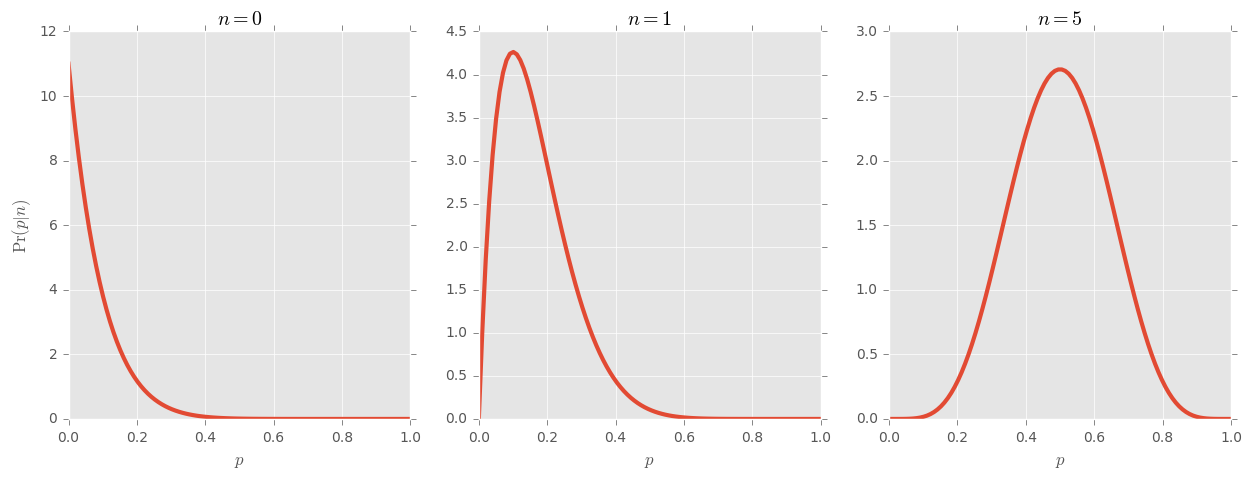# Enter: QInfer¶

For more complicated models, such an analytic solution is not obtainable and we must turn to numerical algorithms. The algorithm we use is called sequential Monte Carlo or the particle filter. We begin by drawing $k$ samples $\{p_i\}$ from the prior and approximating it as $$\operatorname{Pr}(p) = \frac{1}{k}\sum_{i=1}^k \delta(p-p_i).$$ The samples are called particles. Each particle is associated a weight $w_i$ which is conventionally set to be $1/k$ initially. The weights are updated via Bayes rule as follows: $$w_i(n) \mapsto \Pr(n|p_i)/k.$$ The set of weights $\{w_i(n)\}$ are not normalized so we make one final assignment $$w_i(n) \mapsto \frac{w_i(n)}{\sum_j w_j(n)}.$$ Then the posterior is approximated by $$\operatorname{Pr}(p|n) = \sum_{i=1}^k w_i(n) \delta(p-p_i).$$

That's it; that's the basics!

QInfer is numerical algorithm to approximate Bayesian inference. Beyond flipping coins, we treat Bayesian inference as the problem of inferring a set of parameters $\boldsymbol{x} \in \mathbb R^n$ from data conditioned on an experiment. That is, we want to know what $\Pr(\boldsymbol{x}|d,e)$ is. Data are generated from a distribution given by a physical model that is known: $\Pr(d|\boldsymbol{x},e)$. In order to compute this, we also need a prior $\Pr(\boldsymbol{x})$.

QInfer mirrors these objects with the following classes

• qinfer.Distribution Represents drawing samples from a prior distribution.
• qinfer.Model Represents evaluation of the likelihood function.
• qinfer.SMCUpdater Represents Bayesian updating via the particle filtering algorithm.

QInfer implements many commonly used distributions and models used in quantum information science, including

• quantum state tomography
• phase estimation
• randomized benchmarking

We'll jump right in and learn how to use QInfer to solve problems in these domains.

# Quantum state tomography¶

To define the model, we start with a basis for traceless Hermitian operators $\{B_j\}_{j=1}^{d^2-1}$. In the case of a qubit, this could be the basis of Pauli matrices, for example. Then, any state $\rho$ can be written $$\rho = \frac{I}{d}+\sum_{j=1}^{d^2-1} \theta_j B_j,$$ for some vector of parameters $\vec\theta$. These parameters must be constrained such that $\rho\geq0$. Defining a basis turns the quantum problem into an effectively classical one.

QInfer's TomographyModel abstracts many of the implementation details of this problem, exposing tomographic models and estimates in terms of QuTip objects, which you will learn about next lecture. For now, the added functionality provided by QuTip will be hidden.

Tomography support in QInfer requires one of the bases mentioned above in order to parameterize the state. Many common choices of basis are included as TomographyBasis objects, such as the Pauli or Gell-Mann bases.

Let us look at an example for a single qubit. Take the Pauli basis: $$\{\sigma_x/\sqrt{2}, \sigma_y/\sqrt{2}, \sigma_z/\sqrt{2}\}.$$ As written above, any state can now be describe by 3 real numbers $(x,y,z)$, often called the Bloch vector, as follows: $$\rho = 1/\sqrt{2}\left(\frac{I}{\sqrt{2}} + x\sigma_x +y\sigma_y + z\sigma_z\right).$$

Many of the most commonly used priors are already implemented as a Distribution. Here we will focus on the GinibreDistribution because is most closely resembles the "flat" prior.

In :
basis = qi.tomography.pauli_basis(1)
prior = qi.tomography.distributions.GinibreDistribution(basis)


In doing simulations, we need to select a "true" state. We can use our prior distribution to select an unbiased "random" state for us.

In :
true_state = prior.sample()


As a qubit is point in a 3 dimensional space, for visualization purposes, it is more convenient to consider a rebit; a qubit constrained to the $X$-$Z$ plane. The Tomography module implements the visualization of distributions through the methods plot_rebit_prior and plot_rebit_posterior.

In :
qi.tomography.plot_rebit_prior(prior, rebit_axes=[1, 3], true_state=true_state, true_size=500)

C:\Users\cgranade\Anaconda2\lib\site-packages\matplotlib\__init__.py:898: UserWarning: axes.color_cycle is deprecated and replaced with axes.prop_cycle; please use the latter.
warnings.warn(self.msg_depr % (key, alt_key))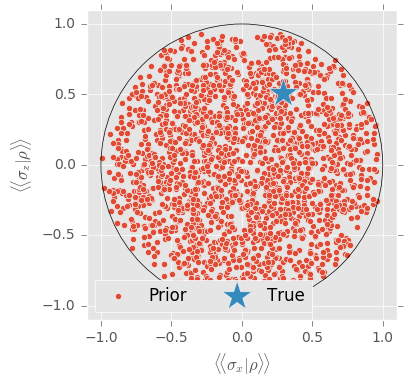Brief side note: Note that this projects the qubit down to a specified pair of axes. To get a better idea of what the GinibreDistribution "looks like" we can use the GinibreReditDistribution which does not project down from another axis.

In :
qi.tomography.plot_rebit_prior(
qi.tomography.distributions.GinibreReditDistribution(basis),
rebit_axes=[1, 3]
)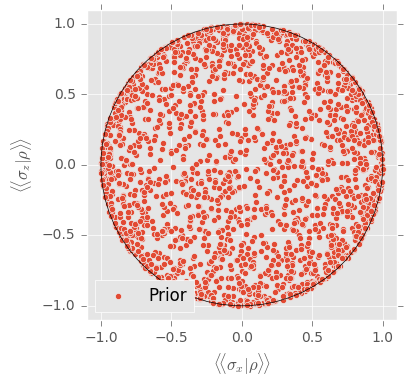In the simplest case, we can consider two-outcome measurements represented by the pair $\{E,I-E\}$. The Born rule defines the likelihood function $$\Pr(E|\rho)=\operatorname{Tr}(\rho E).$$ For multiple measurements, we simply iterate. For many trials of the same measurement, we can use a derived model as discussed below. The actual measurement performed ($E$) is specified as set of experiment parameters, which we will let QInfer automatically choose below.

In :
model = qi.tomography.TomographyModel(basis)


The core method in QInfer is the SMCUpdater which performs the Bayesian updates. It is instantiated with a prior and a model. It internally holds a representation of the current distribution as a set of weighted particles, the number of which controls the approximation and must be specified when instantiating.

Note that the complexity of computing the posterior is roughly linear in the number of particles.

In :
n_particles = 10000
updater = qi.SMCUpdater(model, n_particles, prior)


For simulations, common randomized measurement choices are already implemented. For example, RandomPauliHeuristic chooses random Pauli measurements for qubit tomography.

In :
hueristic = qi.tomography.RandomPauliHeuristic(updater)


Now we simulate experiments and update our prior knowledge using QInfer.

In :
n_measurements = 100

for idx_meas in range(n_measurements):
expparams = hueristic()
datum = model.simulate_experiment(true_state, expparams)
updater.update(datum, expparams)

c:\users\cgranade\dropbox\software-projects\python-qinfer\src\qinfer\utils.py:268: ApproximationWarning: Numerical error in covariance estimation causing positive semidefinite violation.
warnings.warn('Numerical error in covariance estimation causing positive semidefinite violation.', ApproximationWarning)


Finally, we plot the posterior, its mean and a 95% credible ellipse.

In :
qi.tomography.plot_rebit_posterior(updater,
true_state=true_state, rebit_axes=[1, 3],
legend=False, region_est_method='cov'
)
plt.legend(ncol=1, numpoints=1, scatterpoints=1, bbox_to_anchor=(1.9, 0.5), loc='right')

Out:
<matplotlib.legend.Legend at 0x10fc7978>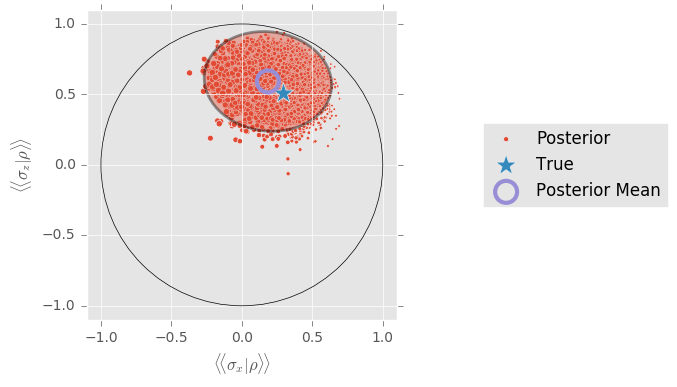# Phase estimation¶

Consider the following setup. The qubit begins in the state $|+\rangle = |0\rangle + |1\rangle$ and is subject to the gate $U(t) = \exp(- j \omega t \sigma_z /2)$, where $t$ is time and $\omega$ is a parameter which controls how strongly the qubit is coupled to some external degrees of freedom. Thinking about the Bloch sphere, the state starts pointing along the positive $x$-axis and rotates in the $x$-$y$ plane. The angle of rotation, which we call the phase, is $\theta = \omega t$. That is, the state becomes $$U(t)|+\rangle = \cos(\omega t /2 ) |+\rangle - j \sin(\omega t /2) |-\rangle.$$ Finally, we measure in the basis $\{|+\rangle,|-\rangle\}$, for which the probability is $$\Pr(+|\omega,t) = \cos^2(\omega t/2).$$

In :
omega_max = 100
true_omega = 23.3
ts = np.linspace(1, 101,25) / (2 * omega_max)

ps = np.cos(true_omega * ts / 2) ** 2

n_counts = 10
counts = np.random.binomial(n_counts, ps)

plt.figure(figsize = (10,5))

plt.plot(ts,ps, linewidth = 3, clip_on=False)

plt.errorbar(ts,counts/n_counts,np.sqrt(ps*(1-ps)/n_counts),
fmt='o',markersize=10, capsize=3, capthick = 3, elinewidth=3, clip_on=False)

plt.ylim([0,1])
plt.ylabel("Probability of '+'")
plt.xlabel("$t$")

Out:
<matplotlib.text.Text at 0x112eb898>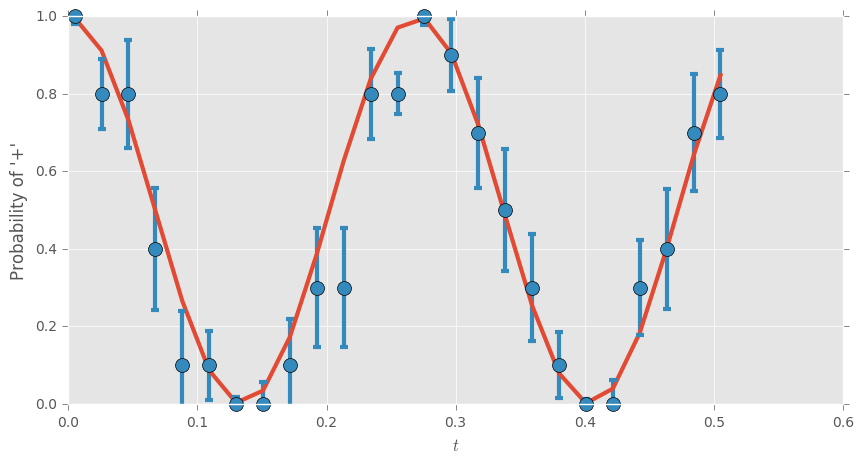The canonical method to estimate the frequency of a sampled signal is via the Fourier transform. This is implemented by the module numpy.fft.

In :
pow_spec = np.abs(np.fft.rfft(counts - np.mean(counts), n=1024)) ** 2

omega = np.linspace(0, np.pi * omega_max / 2, pow_spec.size)

plt.figure(figsize=(10, 5))

plt.plot(omega, pow_spec, linewidth=3, clip_on=False)

plt.ylabel("Power Spectrum")
plt.xlabel(r"$\omega$")

ylim = plt.ylim(ymin=0)
plt.vlines(true_omega, *ylim)
plt.ylim(*ylim);

max_spec = np.argmax(pow_spec) * np.pi * omega_max / 1024
print("The max of the spectrum is {:.2f}.".format(max_spec))

The max of the spectrum is 25.46.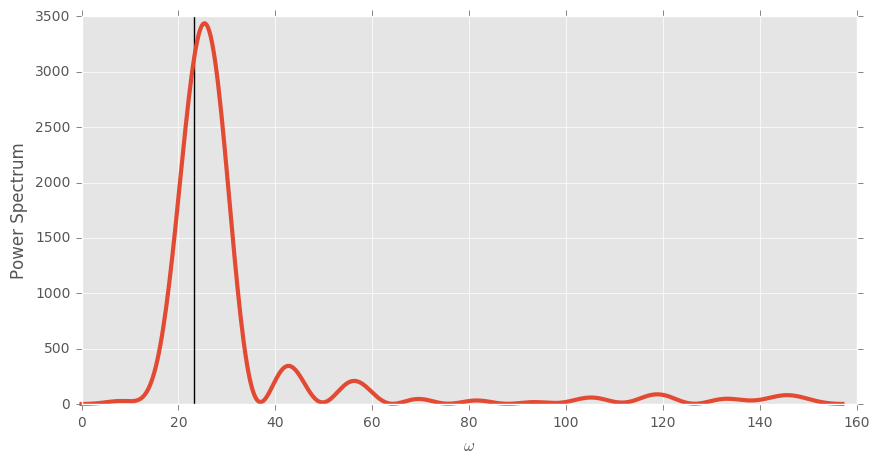The model for this problem, $\Pr(+|\omega,t) = \cos^2(\omega t/2)$, is implemented in QInfer through SimplePrecessionModel. Note that this model provides the likelihood function for a single trial. For the multiple trials above, we need to decorate the model with BinomialModel.

We'll use a uniform prior to specify our initial knowledge. This is implemented by distributions.UniformDistribution and takes as input a range, in this case [0,omega_max].

In :
model = qi.BinomialModel(qi.SimplePrecessionModel())
prior = qi.distributions.UniformDistribution([0, omega_max])
updater = qi.SMCUpdater(model, 10000, prior)


Since each data point is a number of counts at a specific evolution time, we update our distribution by iterating over the number of different evolution times chosen.

In :
for idx_exp in range(25):
expparams = np.array([(ts[idx_exp], n_counts)], dtype=model.expparams_dtype)
datum = n_counts - counts[idx_exp]
updater.update(datum, expparams)


Now the updater represents the posterior distribution. A convenient estimate of $\omega$ is the mean of this distribution. For distributions of single parameters, plotting the full distribution is also useful. QInfer's plot_posterior_marginal performs a smoothed kernel estimate of the distribution from the current state of the particle filter.

In :
plt.figure(figsize=(10, 5))

updater.plot_posterior_marginal(smoothing=.025, other_plot_args={'linewidth': 3})

ylim = plt.ylim(ymin=0)
plt.vlines(true_omega, *ylim)

print("The mean of the distribution is {:.2f}.".format(updater.est_mean()))

The mean of the distribution is 23.07.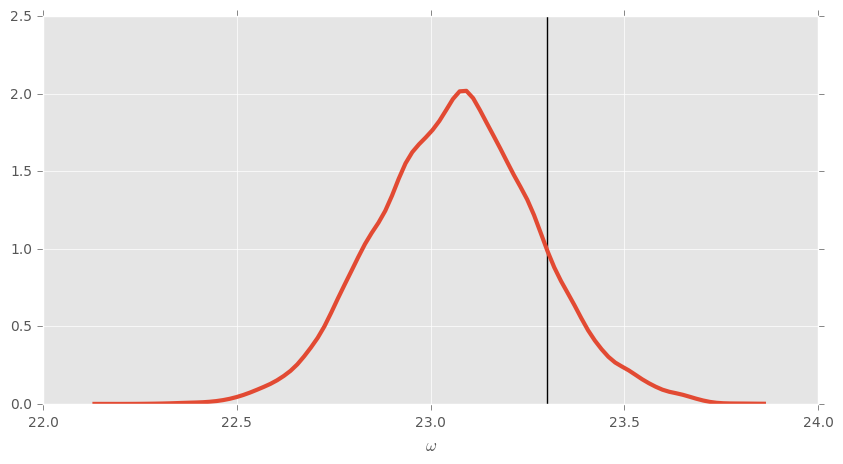Another useful way to visualize the different in these estimates is to plot the predictive probability curve for all $t$.

In :
plt.figure(figsize=(10, 5))

plt.plot(ts, ps, linewidth=3, label='True')

plt.plot(ts,
np.cos(
updater.est_mean() * ts / 2
) ** 2,
linewidth=3, label="Bayes"
)

plt.plot(ts,
np.cos(
np.argmax(pow_spec) * np.pi * omega_max / 1024 * ts / 2
) ** 2,
linewidth=3, label="Fourier"
)

plt.ylim([0, 1])
plt.ylabel("Probability of '+'")
plt.xlabel("$t$")

plt.legend(loc=4)

Out:
<matplotlib.legend.Legend at 0x10de5fd0>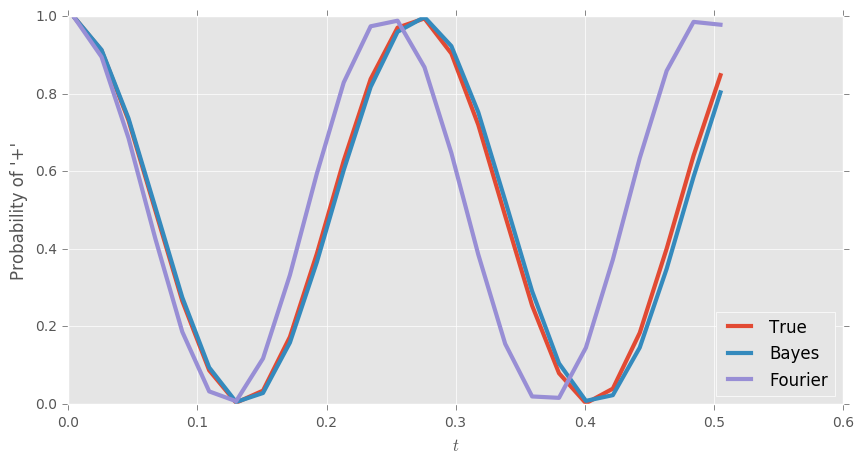# Randomized Benchmarking¶

In recent years, randomized benchmarking (RB) has reached a critical role in evaluating candidate quantum information processing systems. By using random sequences of gates drawn from the Clifford group, RB provides a likelihood function that depends on the fidelity with which each Clifford group element is implemented, allowing for estimates of that fidelity to be drawn from experimental data.

In particular, suppose that each gate is implemented with fidelity $F$, and consider a fixed initial state and measurement. Then, the survival probability over sequences of length $m$ is given by Magesan, Gambetta and Emerson(2011): $$\Pr(+ | p, A, B; m) = A p^m + B,$$ where $p = (d F - 1) / (d - 1)$, $d$ is the dimension of the system under consideration, and where $A$ and $B$ describe the state preparation and measurement (SPAM) errors. Learning the model $\boldsymbol{x} = (p, A, B)$ thus provides an estimate of the fidelity of interest $F$.

The likelihood function for randomized benchmarking is extremely simple, and requires only scalar arithmetic to compute, making it especially useful for avoiding the computational overhead typically required to characterize large quantum systems with classical resources.

QInfer supports RB experiments through the RandomizedBenchmarkingModel class. For common priors, QInfer also provides a simplified interface, simple_est_rb, that reports the mean and covariance over an RB model given experimental data.

In :
p, A, B = 0.95, 0.5, 0.5
ms = np.linspace(1, 101, 10).astype(int)

F = A * p ** ms + B

n_counts = 100
counts = np.random.binomial(n_counts, F)

plt.figure(figsize=(10, 5))

plt.plot(ms, F, linewidth=3, clip_on=False)
plt.errorbar(ms, counts / n_counts, np.sqrt(F * (1 - F) / n_counts),
fmt='o', markersize=10,
capsize=3, capthick=3, elinewidth=3, clip_on=False)

plt.ylabel("Probability of '+'")
plt.xlabel("$m$")

Out:
<matplotlib.text.Text at 0x110d7710>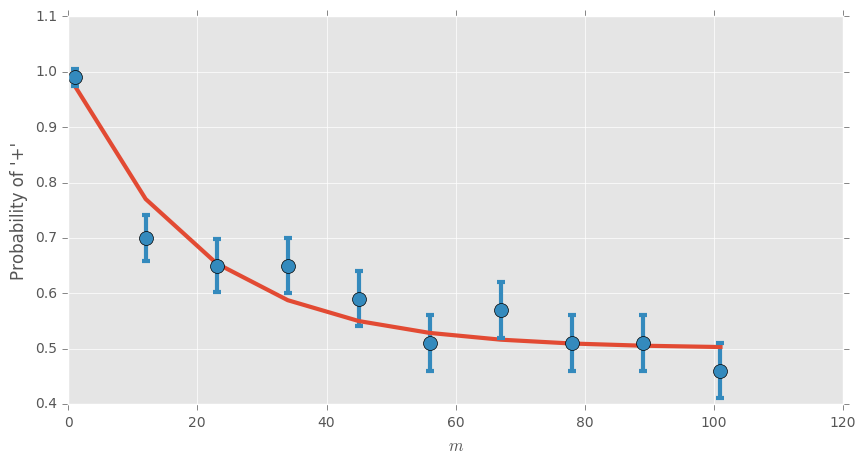The standard method to estimate $p, A$ and $B$ from this data is least squares fitting. This can be achieved by SciPy's method optimize.least_squares.

In :
def func(x):
p, A, B = x, x, x
return A * p ** ms + B ** 2 - counts / n_counts

lstsq = sp.optimize.leastsq(func, np.array([.95, .25, .25]))

print("Least squares solution for (p,A,B) is ({:.4f},{:.3f},{:.3f}).".format(*lstsq))

Least squares solution for (p,A,B) is (0.9504,0.478,0.713).


For some common problems, such as this one, QInfer implements functions which automatically make some choices and perform the updating. For this problem, the function we want is simple_est_rb.

In :
data = np.column_stack([counts, ms, n_counts * np.ones_like(counts)])

mean, cov, extra = qi.simple_est_rb(data, return_all=True, n_particles=12000, p_min=0.8)

print("Least squares solution for (p,A,B) is ({:.4f},{:.3f},{:.3f}).".format(*mean))

Least squares solution for (p,A,B) is (0.9470,0.489,0.495).


Finally, we plot the posterior. We cannot do so for the full distribution over $(p,A,B)$, but we can plot the marginal distributions. This is done with the keyword argument idx_param, which defaults to 0, $p$.

In :
plt.figure(figsize=(10, 5))

extra['updater'].plot_posterior_marginal(smoothing=.01, range_max=1, other_plot_args={'linewidth': 3})
plt.xlim(xmax=1)
plt.vlines(p, *plt.ylim())

Out:
<matplotlib.collections.LineCollection at 0x11191cf8>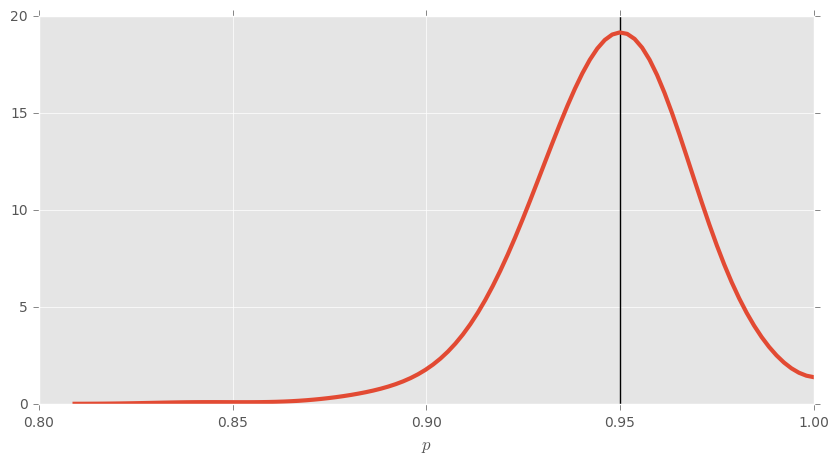# Custom Models in QInfer¶

Now we will demonstrate how to create a custom Model in QInfer. To this end we return to the coin flipping example.

In :
# We create a model which is abstracted by the Model class, which already implements common methods
# among models and templates what such a class needs to look like

class CoinFlipsModel(qi.FiniteOutcomeModel):

# This function is called when an instance of this class is instantiated.
def __init__(self):
# Make sure all the necessary functionality in the Model class is brought over.
super(CoinFlipsModel, self).__init__()

# The following are functions that are required for the functionality of QInfer.

@property
def n_modelparams(self):
# The dimension of the parameter space.
return 1 # In this case, it is just the bias p.

@property
def expparams_dtype(self):
# many models will have complicated experiment design descriptions. Here, it is just the number of flips.
return [('N', int)] # The number of flips is an integer.

@property
def is_n_outcomes_constant(self):
# We tell QInfer that the number of outcomes
# depends on which experiment we want to perform.
return False

def n_outcomes(self, expparams):
# The number of outcomes are needed to numerically simulate experiments.
return expparams + 1 # the number of flips + 1

def are_models_valid(self, modelparams):
# Make sure any boundaries in the parameter space are respected.
# In this case, the modelparams array is a bias, which must be between 0 and 1.
return np.logical_or(modelparams >= 0, modelparams <= 1)[:, 0]

def likelihood(self, outcomes, modelparams, expparams):
# Finally, we calculate the likelihood function
# Again, call some necessary internal functions.
super(CoinFlipsModel,self).likelihood(outcomes, modelparams, expparams)

# The tensor storing the values of the likelihood has the following expected shape.
like = np.zeros([outcomes.shape, modelparams.shape, 1])

# scipy.stats.binom can vectorize over one of its arguments so we'll have to loop over the other
for idx in range(outcomes.shape):
like[idx] = sp.stats.binom.pmf(outcomes[idx], expparams['N'], modelparams)

return like


Now that is a bit overkill for such a simple problem, but the functionality is there really for the more "interesting" models, such as those we encountered above. But we can see that calling model.likelihood gives the correct results.

In :
model = CoinFlipsModel() # This instantiates the model we just defined.

# Recall that model.expparams_dtype was int and we defined N to be an integer above.
expparams = np.array([(N,)], dtype=model.expparams_dtype)
# In the model we asked for outcomes.shape, so we have to define it as an object with a shape!
outcome = np.array()

fig, axarr = plt.subplots(1, 3, figsize=(15, 5))
# Note that modelparams are expected to have at least 2D shape;
# indexing by None adds an axis to the (100,) array we defined above for p.
p = np.linspace(0, 1, 100)
axarr.plot(p, model.likelihood(outcome, p[:, None], expparams)[0, :, 0], linewidth=3)
axarr.set_xlabel("$p$")
axarr.set_ylabel("$\operatorname{Pr}(n|p)$")
axarr.set_title("$n=0$")

outcome = np.array()
axarr.plot(p, model.likelihood(outcome, p[:, None], expparams)[0, :, 0], linewidth=3)
axarr.set_xlabel("$p$")
axarr.set_title("$n=1$")

outcome = np.array()
axarr.plot(p, model.likelihood(outcome, p[:, None], expparams)[0, :, 0], linewidth=3)
axarr.set_xlabel("$p$")
axarr.set_title("$n=5$")

Out:
<matplotlib.text.Text at 0x123e8208>As a side note, we could have also taken a hybrid approach. That is, we could create a model for single coin flip and then decorate that with BinomialModel.

In :
# Much of this will be the same as above

class CoinFlipModel(qi.FiniteOutcomeModel):

def __init__(self):
super(CoinFlipModel, self).__init__()

@property
def n_modelparams(self):
return 1

@property
def expparams_dtype(self):
return []

@property
def is_n_outcomes_constant(self):
return True

def n_outcomes(self, expparams):
return 2

def are_models_valid(self, modelparams):
return np.logical_or(modelparams >= 0, modelparams <= 1)[:, 0]

def likelihood(self, outcomes, modelparams, expparams):
super(CoinFlipModel,self).likelihood(outcomes, modelparams, expparams)

pr0 = modelparams[:,np.newaxis]

return qi.FiniteOutcomeModel.pr0_to_likelihood_array(outcomes, pr0)


And, just to verify, let's make sure it gives the same answer.

In :
dec_model = qi.BinomialModel(CoinFlipModel())

# Recall that model.expparams_dtype was int and we defined N to be an integer above.
dec_expparams = np.array([(N,)], dtype=dec_model.expparams_dtype)

# In the model we asked for outcomes.shape, so we have to define it as an object with a shape!
outcome = np.array([N - 0])

fig, axarr = plt.subplots(1, 3, figsize=(15, 5))
# Note that modelparams are expected to have at least 2D shape;
# indexing by None adds an axis to the (100,) array we defined above for p.
p = np.linspace(0, 1, 100)
axarr.plot(p, dec_model.likelihood(outcome, p[:, None], dec_expparams)[0, :, 0], linewidth=3)
axarr.set_xlabel("$p$")
axarr.set_ylabel("$\operatorname{Pr}(n|p)$")
axarr.set_title("$n=0$")

outcome = np.array([N-1])
axarr.plot(p, dec_model.likelihood(outcome, p[:, None], dec_expparams)[0, :, 0], linewidth=3)
axarr.set_xlabel("$p$")
axarr.set_title("$n=1$")

outcome = np.array([N-5])
axarr.plot(p, dec_model.likelihood(outcome, p[:, None], dec_expparams)[0, :, 0], linewidth=3)
axarr.set_xlabel("$p$")
axarr.set_title("$n=5$")

Out:
<matplotlib.text.Text at 0x12870b00>As a final check, let's make sure this leads to an approximately correct posterior using QInfer. First, we need a distribution.

In :
# This instantiates the built-in uniform prior over the interval [0, 1].
prior = qi.UniformDistribution([0, 1])


The last thing to do is define an SMCUpdater which implements the sequential Monte Carlo algorithm. An SMCUpdater takes a model and prior and then its update function takes data and experiment parameters to produce the posterior distribution. The number of particles used controls the approximation.

In :
# The number of particles we will use.
n_particles = 10000

# Instantiates an updater, takes the model and prior we defined above.
updater = qi.SMCUpdater(model, n_particles, prior)


OK, now we can plug in some data and see how well it does.

In :
# In the model we asked for outcomes.shape, so we have to define it as an object with a shape!
outcome = np.array()

# Call the update function to change the state of the updater, now it stores the posterior.
updater.update(outcome, expparams)

plt.figure(figsize=(10, 5))

plt.plot(p, posterior(p, 5, N), label = 'True Posterior', linewidth = 3)
updater.plot_posterior_marginal(smoothing=0.005, other_plot_args={'label': 'QInfer approximation', 'linewidth':3})

plt.xlabel("$p$")
plt.ylabel("$\operatorname{Pr}(n|p)$")

plt.legend()

Out:
<matplotlib.legend.Legend at 0x12b81a20>